Courses

# NCERT Exemplar - Classification of Elements & Periodicity in Properties Notes | EduRev

## JEE : NCERT Exemplar - Classification of Elements & Periodicity in Properties Notes | EduRev

The document NCERT Exemplar - Classification of Elements & Periodicity in Properties Notes | EduRev is a part of the JEE Course JEE Revision Notes.
All you need of JEE at this link: JEE

MULTIPLE CHOICE QUESTIONS (TYPE - I)

Q.1. Consider the isoelectronic species, Na+, Mg2+, F and O2–.  The correct order of increasing length of their radii is ______.
(i) F- < O2– < Mg2+ < Na+
(ii) Mg2+ < Na+ < F < O2–
(iii) O2– < F < Na+ < Mg2+
(iv) O2– < F <  Mg2+ < Na
+
Ans. (ii)
Solution.
All of them are isoelectronic species. They have same number of electrons (10). Their radii would be different because of their different nuclear charges. The cation with the greater positive charge will have a smaller radius because of the greater attraction of the electrons to the nucleus. Anion with the greater negative charge will have the larger radius. In this case, the net repulsion of the electrons will outweigh the nuclear charge and the ion will expand in size.

Q.2. Which of the following is not an actinoid?
(i) Curium (Z  = 96)
(ii) Californium (Z = 98)
(iii) Uranium (Z = 92)
(iv) Terbium (Z = 65)
Ans. (iv)
Solution.
Actinoids are elements with Z= 90-103. They are characterised by the outer electronic configuration (n-2) f-14 (n-1) dns2. The last electron added to each element is filled in f-orbital. Hence Terbium (Z = 65) is not an actinoid. It is a lanthanoid.

Q.3. The order of screening effect of electrons of s, p, d and f orbitals of a given shell of an atom on its outer shell electrons is:
(i) s > p > d > f
(ii) f > d > p > s
(iii) p < d < s > f
(iv) f > p > s > d
Ans. (i)
Solution.
The effective nuclear charge experienced by a valence electron in an atom will be less than the actual charge on the nucleus because of ‘‘shielding” or “screening” of the valence electron from the nucleus by the intervening core electrons. For example, the 2s electron in lithium is shielded from the nucleus by the inner core of 1s electrons.

Q.4. The first ionisation enthalpies of Na, Mg, Al and Si are in the order:
(i) Na < Mg > Al < Si
(ii) Na > Mg > Al > Si
iii) Na < Mg < Al < Si
(iv) Na > Mg > Al < Si
Ans. (i)
Solution.
The electronic configurations of Na and Mg are:
Na (11): [Ne] 3s1 and Mg (12): [Ne] 3sIn both the atoms, the electron is to be removed from 3s-orbital but nuclear charge in Na is less than Mg. Thus, ionisation energy of Na is less than Mg (Na < Mg).
The electronic configurations of Mg and Al are:
Mg: [Ne] 3s2; Al: [Ne] 3s2 3pl
In Mg, the electron is to be removed from 3s-orbital while in Al, it is to be removed from
3p-orbital. Since it is easier to remove an electron from 3p-orbital in comparison to 3s
orbital, the ionization enthalpy of Mg is higher than Al (Mg > Al).
The electronic configurations of Al and Si are:
Al (13): [Ne] 3s2 3p1 Si (14): [Ne] 3s2 3p2
In both the atoms, the electron is to be removed from 3p-orbital but nuclear charge in Si is more than Al. Thus, ionisation enthalpy of Al is less than Si

Q.5. The electronic configuration of gadolinium (Atomic number 64) is
(i) [Xe] 4f3 5d5 6s2
(ii) [Xe] 4f7 5d2 6s1
(iii) [Xe] 4f7 5d1 6s2
(iv) [Xe] 4f8 5d6 6s2
Ans. (iii)
The electronic configuration of La (Z = 57) is [Xe] 5dl 6s2. Therefore, further addition of electrons occurs in the lower energy 4f-orbital till it is exactly half-filled at Eu (Z = 63) Thus, the electronic configuration of Eu is [Xe] 4f7 6s2. Thereafter, addition of next electron does not occur in the more stable exactly half-filled 4f7 shell but occurs in the little higher energy 5d-orbital. Thus, the electronic configuration of Gd (Z = 64) is [Xe] 4f7 5d6s2.

Q.6. The statement that is not correct for periodic classification of elements is:
(i) The properties of elements are periodic function of their atomic numbers. (ii) Non metallic elements are less in number than metallic elements.
(iii) For transition elements, the 3d-orbitals are filled with electrons after 3p-orbitals and before 4s-orbitals.
(iv) The first ionisation enthalpies of elements generally increase with increase in atomic number as we go along a period.
Ans. (iii)
Solution.
For transition elements, the 3d-orbitals are filled with electrons after 3p and 4s-orbitals and before 4p-orbitals. The order of filling the orbitals is:
1s 2s 2p 3s 3p 4s 3d 4p 5s 4d 5p 6s …

Q.7. Among halogens, the correct order of amount of energy released in electron gain (electron gain enthalpy) is:
(i) F > Cl > Br > I
(ii) F < Cl < Br < I
(iii) F < Cl > Br > I
(iv) F < Cl < Br < I
Ans. (iii)
Chlorine has higher electron gain enthalpy than fluorine. This is due to small size of fluorine atom, i.e., the electron density is high which resists the addition of an electron (F < Cl).
In general, electron gain enthalpy decreases as atomic size increases. Thus, electron gain enthalpy follows the order:
Cl > Br > I

Q.8. The period number in the long form of the periodic table is equal to
(i) magnetic quantum number of any element of the period.
(ii) atomic number of any element of the period.
(iii) maximum Principal quantum number of any element of the period.
(iv) maximum Azimuthal quantum number of any element of the period.
Ans. (iii)
Solution.
Since each period starts with the filling of electrons in a new principal quantum number, therefore, the period number in the long form of the periodic table refers to the maximum principal quantum number of any element in the period.
Period number = maximum n of any element
(where, n = principal quantum number).

Q.9. The elements in which electrons are progressively filled in 4f-orbital are called
(i) actinoids
(ii) transition elements
(iii) lanthanoids
(iv) halogens
Ans. (iii)
Solution.
The sixth period (n = 6) contains 32 elements and successive electrons enter 6s, 4f, 5d and 6p orbitals, in the order of filling up of the 4f orbitals begins with cerium (Z = 58) and ends at lutetium (Z = 71) to give the 4f-inner transition series which is called the lanthanoid series.

Q.10. Which of the following is the correct order of size of the given species:
(i) I > I > I+
(ii) I+ > I > I
(iii) I > I+ > I
(iv) I > I > I+
Ans. (iv)
Solution.
A cation is smaller than its parent atom because it has fewer electrons while its nuclear charge remains the same. The size of an anion will be larger than that of the parent atom because the addition of one or more electrons would result in increased repulsion among the electrons and a decrease in effective nuclear charge.

Q.11. The formation of the oxide ion, O2 (g), from oxygen atom requires first an exothermic and then an endothermic step as shown below:
O (g) + e → O (g) ; ∆ HΘ =  - 141 kJ mol-1
O (g) + e → O2– (g); ∆ HΘ =  + 780 kJ mol-1
Thus process of formation of O2– in gas phase is unfavourable even though O2– is isoelectronic with neon. It is due to the fact that,
(i) oxygen is more electronegative.
(ii) addition of electron in oxygen results in larger size of the ion.
(iii) electron repulsion outweighs the stability gained by achieving noble gas configuration.
(iv) O ion has comparatively smaller size than oxygen atom.
Ans. (iii)
Solution.
When an electron is added to O atom to form O- ion, energy is released. Thus, the first electron gain enthalpy of O is negative. On the other hand, when an electron is added to O- ion to form O2-ion, energy has to be given out in order to overcome the strong electronic repulsions. Thus the second electron gain enthalpy of O is positive.

Q.12. Comprehension given below is followed by some multiple choice questions. Each question has one correct option. Choose the correct option.
In the modern periodic table, elements are arranged in order of increasing atomic numbers which is related to the electronic configuration. Depending upon the type of orbitals receiving the last electron, the elements in the periodic table have been divided into four blocks, viz, s, p, d and f. The modern periodic table consists of 7 periods and 18 groups. Each period begins with the filling of a new energy shell. In accordance with the Arfbau principle, the seven periods (1 to 7) have 2, 8, 8, 18, 18, 32 and 32 elements respectively. The seventh period is still incomplete. To avoid the periodic table being too long, the two series of f-block elements, called lanthanoids and actinoids are placed at the bottom of the main body of the periodic table.
(a) The element with atomic number 57 belongs to
(i) s-block
(ii) p-block
(iii) d-block
(iv) f-block

Ans. (iii)
Solution.
Lanthanoids which are characterised by the filling of 4f-orbitals, are the elements following lanthanum from 58Ce to 71Lu. Actinoids characterised by filling of 5f-orbitals, are the elements following actinium from 90Th to 103Lr. Characteristic outer electronic configuration is (n - 2)f14 (n - 1)d0-1ns2.

(b) The last element of the p-block in 6th period is represented by the outermost electronic configuration.
(i) 7s2 7p6
(ii) 5f14 6d10 7s2 7p0
(iii) 4f14 5d10 6s2 6p6
(iv) 4f14 5d10 6s2 6p4
Ans. (iii)
Solution.
The sixth period (n = 6) contains 32 elements and successive electrons enter 6s, 4f, 5d and 6p orbitals. The last element in the p-block in 6th period is 86Rn.

(c) Which of the elements whose atomic numbers are given below, cannot be accommodated in the present set up of the long form of the periodic table?
(i) 107
(ii) 118
(iii) 126
(iv) 102
Ans. (iii)
Solution.
The present set up of the long form of the periodic table has seven periods and four blocks. So, the maximum number of elements according to Aufbau principle which can be accommodated are 118.

(d) The electronic configuration of the element which is just above the element with atomic number 43 in the same group is ______.
(i) 1s2 2s2 2p6 3s2 3p6 3d5 4s2
(ii) 1s2 2s2 2p6 3s2 3p6 3d5 4s2 4p6
(iii) 1s2 2s2 2p6 3s2 3p6 3d6 4s2
(iv) 1s2 2s2 2p6 3s2 3p6 3d7 4s2
Ans. (i)
Solution.
The atomic number of the element which lies just above the element with atomic number 43 is 25 (Mn). Its electronic configuration is 1s2 2s2 2p6 3s2 3p6 3d5 4s2.

(e) The elements with atomic numbers 35, 53 and 85 are all ______.
(i) noble gases
(ii) halogens
(iii) heavy metals
(iv) light metals
Ans. (ii)
Solution.
The elements with atomic numbers 35, 53 and 85 lie in a group before noble gases i.e., halogens (group 17). Thus, the elements with atomic numbers 35, 53 and 85 are halogens.

Q.13. Electronic configurations of four elements A, B, C and D are given below:
(A) 1s2 2s2 2p6
(B)  1s2 2s2 2p4
(C) 1s2 2s2 2p6 3s1
(D)  1s2 2s2 2p5
Which of the following is the correct order of increasing tendency to gain electron :
(i) A < C < B < D
(ii) A < B < C < D
(iii) D < B < C < A
(iv) D < A < B < C

Ans. (i)
A – 1s2 2s2 2p6 – Noble gas configuration
B -1s2 2s2 2p4 – 2 electrons short of stable configuration
C – 1s2 2s2 2p6 3.?1 – Requires one electron to complete 5-orbital
D -1s2 2s2 2p5 – Requires one electron to attain noble gas configuration

• Noble gases have no tendency to gain electrons since all their orbitals are completely filled. Thus, element A has the least electron gain enthalpy.
• Since element D has one electron less and element B has two electrons less than the corresponding noble gas configuration, hence, element D has the highest electron gain enthalpy followed by element B.
• Since, element C has one electron in the 5-orbital and hence needs one more electron to complete it, therefore, electron gain enthalpy of C is less than that of element B. Combining all the facts given above, the electron gain enthalpies of the four elements increase in the order A < C < B < D.

MULTIPLE CHOICE QUESTIONS (TYPE - II)

In the following questions two or more options may be correct.
Q.14. Which of the following elements can show covalency greater than 4?
(i) Be
(ii) P
(iii) S
(iv) B
Ans. (ii) and (iii)
Solution.
P and S have d-orbitals in their valence shell and therefore, can accommodate more than 8. electrons in their respective valence shells. Hence they show covalency more than 4.

Q.15. Those elements impart colour to the flame on heating in it, the atoms of which require low energy for the ionisation (i.e., absorb energy in the visible region of spectrum). The elements of which of the following groups will impart colour to the flame?
(i) 2
(ii) 13
(iii) 1
(iv) 17
Ans. (i) and (iii)
Solution.
The elements of group 1 (alkali metals) and group 2 (alkaline earth metals) have low ionization enthalpies. Therefore, they impart colour to flame.

Q.16. Which of the following sequences contain atomic numbers of only representative elements?
(i) 3, 33, 53, 87
(ii) 2, 10, 22, 36
(iii) 7, 17, 25, 37, 48
(iv) 9, 35, 51, 88

Ans. (i) and (iv)
Solution.
Elements of 5 and p-block elements are called representative elements. Elements of f-block (Z=21 – 30; 39 – 48; 57 and 72 – 80; 89 and 104 – 112) are called transition elements while those of f-block (with Z = 58-71 and Z = 90 – 103) are called inner transition elements.
(i) 3 – Group 1, 33 – group 15, 53 – group 17 and 87 – group 1.
(ii) 9 – Group 17, 35 – Group 17, 51 – Group 15, 88 – Group 2.

Q.17. Which of the following elements will gain one electron more readily in comparison to other elements of their group?
(i) S (g)
(ii) Na (g)
(iii) O (g)
(iv) Cl (g)
Ans. (i) and (iv)
Solution.
For many elements energy is released when an electron is added to the atom and the electron gain enthalpy is negative. For example, group 17 elements (the halogens) have very high negative electron gain enthalpies because they can attain stable noble gas electronic configurations by picking up an electron.

Q.18. Which of the following statements are correct?
(i) Helium has the highest first ionisation enthalpy in the periodic table.
(ii) Chlorine has less negative electron gain enthalpy than fluorine.
(iii) Mercury and bromine are liquids at room temperature.
(iv) In any period, atomic radius of alkali metal is the highest.
Ans. (i), (iii) and (iv)
Chlorine has more negative electron gain enthalpy than fluorine due to bigger size and lesser electronic repulsion.

Q.19. Which of the following sets contain only isoelectronic ions?
(i) Zn2+, Ca2+, Ga3+, Al3+
(ii) K+, Ca2+, Sc3+, Cl
(iii) P3, S2, Cl, K+
(iv) Ti4+, Ar, Cr3+, V5+
Ans. (ii) and (iii)
Solution.
(i) Zn2+ (30 – 2 = 28), Ca2+ (20 – 2 = 18), Ga3+ (31-3= 28), Al3+ (13 – 3 = 10) are not isoelectronic.
(ii) K+ (19 – 1 = 18), Ca2+ (20 – 2 = 18), Sc3+ (21 – 3 = 18), Cl (17 + 1 = 18) are isoelectronic.
(iii) P3- (15 + 3 = 18), S2- (16 + 2 = 18), Cl (17 + 1 = 18), K+ (19 – 1 = 18) are isoelectronic.
(iv) Ti4+ (22 – 4 = 18), Ar (18), Cr3+ (24 – 3 = 21), V5+ (23 – 5 = 18) are not isoelectronic.

Q.20. In which of the following options order of arrangement does not agree with the variation of property indicated against it?
(i) Al3+ < Mg2+ < Na+ < F (increasing ionic size)
(ii) B < C < N < O (increasing first ionisation enthalpy)
(iii) I < Br < Cl < F (increasing electron gain enthalpy)
(iv) Li < Na < K < Rb (increasing metallic radius)
Ans. (ii) and (iii)
The ionisation enthalpy of N is higher than that of O due to greater stability of half-filled electronic configuration. Hence (ii) is incorrect.
Again in option (iii), the electron gain enthalpy of F is lower than that of Cl due to small size of F. Hence (iii) is wrong.

Q.21. Which of the following have no unit?
(i) Electronegativity
(ii) Electron gain enthalpy
(iii) Ionisation enthalpy
(iv) Metallic character
Ans. (i) and (iv)
Solution.
A qualitative measure of the ability of an atom in a chemical compound to attract shared electrons to itself is called electronegativity. Unlike ionization enthalpy and electron gain enthalpy, it is not a measureable quantity.

(i) inverse proportion to the effective nuclear charge.
(ii) inverse proportion to the square of effective nuclear charge.
(iii) direct proportion to the screening effect.
(iv) direct proportion to the square of screening effect.
Ans. (i) and (iii)
Solution.
The cation with the greater positive charge will have a smaller radius because of the greater attraction of the electrons to the nucleus. Anion with the greater negative charge will have the larger radius. In this case, the net repulsion of the electrons will outweigh the nuclear charge and the ion will expand in size so the ionic radii vary in inverse proportion to the effective nuclear charge and direct proportion to the screening effect.

Q.23. An element belongs to 3rd period and group-13 of the periodic table. Which of the following properties will be shown by the element?
(i) Good conductor of electricity
(ii) Liquid, metallic
(iii) Solid, metallic
(iv) Solid, non metallic
Ans. (i, iii) The element belonging to 3rd period and 13th group is aluminium which is a metal. Hence, it is solid, metallic and good conductor of electricity.

Q. 24. Explain why the electron gain enthalpy of fluorine is less negative than that of chlorine.
Ans. In fluorine, the new electron to be added goes to 2p-subshell while in chlorine, the added electron goes to 3p-subshell. Since the 2p-subshell is relatively small as compared to 3p-subshell, the added electron in small 2p-subshell experiences strong interelectronic repulsions in comparison to that in 3p-subshell in Cl. As a result, the incoming electron does not feel much attraction from the nucleus and therefore, the electron gain enthalpy of F is less negative than that of Cl.

Q.25. All transition elements are d-block elements, but all d-block elements are not transition elements. Explain.
Ans. All the elements in between s- and p-block, i.e., between group 2 and 13 are called transition elements. Elements in which the last electron enters the d-orbitals of their respective penultimate shells are called d-block elements. According to this definition, Zn, Cd and Hg cannot be regarded as d-block elements because last electron in these elements enters the s-orbital of their outermost shells rather than d-orbital of their penultimate shells. Therefore, these elements should not be regarded as d-block elements. However, the properties of these elements resemble with cf-block elements. Therefore, to make the study of periodic classification of elements more rational, these are studied along with d-block elements. Thus, on the basis of properties, all the transition elements are d-block elements but on the basis of electronic configuration, all d-block elements are not transition elements.

Q.26. Identify the group and valency of the element having atomic number 119. Also predict the outermost electronic configuration and write the general formula of its oxide.
Ans. The present set up of the Long Form of the Periodic Table can accommodate at the maximum 118 elements. After this, according to Aufbau principle, 8.y-orbital should be filled. Therefore, the outer electronic configuration of element having atomic number 119 will be 8s1. Since it has one electron in the outermost s-orbital, its valency will be 1 and it should belong to group 1 along with alkali metals. The general formula of its oxide will be M20, where M represents the element.

Q.27. Ionisation enthalpies of elements of second period are given below: Ionisation enthalpy/ k cal mol–1 :  520, 899, 801, 1086, 1402, 1314, 1681, 2080.
Match the correct enthalpy with the elements and complete the graph given in Figure. Also write symbols of elements with their atomic number.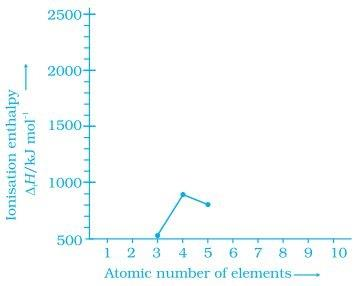Ans.  To match the correct enthalpy with the elements and to complete the graph, the following points are taken into consideration. As we move from left to right across a period, the ionization enthalpy keeps on increasing due to increased nuclear charge and simultaneous decrease in atomic radius. However, there are some exceptions given below

• In spite of increased nuclear charge, the first ionisation enthalpy of B is lower than that of Be. This is due to the presence of fully filled 2s-orbital of Be [1s22s2] which is a stable electronic arrangement. Thus, higher energy is required to knock out the electron from fully filled 2.v-orbital. While B [1s2 2s2 2p1] contains valence electrons in 2s and 2p-orbitals. It can easily lose its one e from 2p-orbital in order to achieve noble gas configuration. Thus, first ionisation enthalpy of B is lower than that of Be.
Since the electrons in 2s-orbital are more tightly held by the nucleus than those present in 2p-orbital, therefore, ionisation enthalpy of B is lower than that of Be.
• The first ionisation enthalpy of N is higher than that of O though the nuclear charge of O is higher than that of N. This is due to the reason that in case of N, the electron is to be removed from a more stable, exactly half-filled electronic configuration (1s2 2s2 21x2ply 2p1z) which is not present in O (1s2 2s2 2p2x 2p1y 2p1z).
Therefore, the first ionisation enthalpy of N is higher than that of O. The symbols of elements along with their atomic numbers are given in the following graph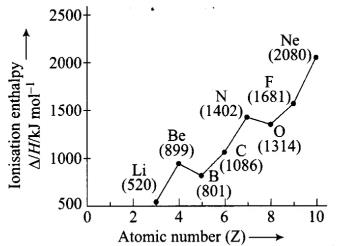Q.28. Among the elements B, Al, C and Si,
(i) which element has the highest first ionisation enthalpy?
(ii) which element has the most metallic character?

Ans. Arranging the elements into different groups and periods:
 Group 13 14 Period 2 B C Period 3 Al Si
• Ionization enthalpy increases along a period and decreases down a group. Therefore, C has the highest first ionization enthalpy.
• Metallic character decreases along a period and increases down a group. Therefore, Al has the most metallic character.

Q.29. Write four characteristic properties of p-block elements.
Ans. The four important characteristic properties of p-block elements are the following:
(a) p-Block elements include both metals and non-metals but the number of non-metals is much higher than that of metals. Further, the metallic character increases from top to bottom within a group and non-metallic character increases from left to right along a period in this block.
(b) Their ionization enthalpies are relatively higher as compared to s-block elements.
(c) They mostly form covalent compounds.
(d) Some of them show more than one (variable) oxidation states in their compounds. Their oxidizing character increases from left to right in a period and reducing character increases from top to bottom in a group.

Q.30. Choose the correct order of atomic radii of fluorine and neon (in pm) out of the options given below and justify your answer.
(i) 72, 160
(ii) 160, 160
(iii) 72, 72
(iv) 160, 72
Ans. (i)
Solution: Atomic radius decreases as we move from left to right in a period in the periodic table. Fluorine has the smallest atomic radius. As we move to neon in the same period, the atomic radius increases as it has van der Waals radius and van der Waals radii are bigger than covalent radii.

Q.31. Illustrate by taking examples of transition elements and non-transition elements that oxidation states of elements are largely based on electronic configuration.
Ans. The oxidation state of an element is based on its electronic configuration. The various oxidation states of a transition metal are due to the involvement of (n-1)d and outer ns electrons in bonding.
For example, Ti (22, electronic configuration [Ar]3d24s2) can show three oxidation states (+2, +3 and +4) in various compounds like Ti02 (+4), Ti20(+3) and TiO (+2).
The non-transition elements, mainly thep-block elements can show a number of oxidation states from +n to (n – 8) where, n is the number of electrons present in the outermost shell. For example, phosphorus can show -3, +3 and +5 oxidation states.
Lower oxidation states are ionic as the atom accepts the electron or electrons to achieve stable configuration while higher oxidation states are achieved by unpairing the paired electrons and shifting the electrons to vacant d-orbital.

Q.32. Nitrogen has positive electron gain enthalpy whereas oxygen has negative. However, oxygen has lower ionisation enthalpy than nitrogen. Explain.
Ans.The outermost electronic configuration of nitrogen is 2s22p1x2p1y2p1z. It is stable because it has exactly half filled 2p-subshell. Therefore, it has no tendency to accept extra electron and energy has to be supplied to add additional electron. Thus, electron gain enthalpy of nitrogen is slightly positive. On the other hand, the outermost electronic configuration of O is 2s22p2x2p1y2p1z. It has higher positive charge (+8) than nitrogen (+7) and lower atomic size than N. Therefore, it has a tendency to accept an extra electron. Thus, electron gain enthalpy of O is negative. However, oxygen has four electrons in the 2p subshell and can lose one electron to acquire stable half filled configuration and therefore, it has low ionization enthalpy. Because of stable configuration of N, it cannot readily lose electron and therefore, its ionization enthalpy is higher than that of O.

Q.33. First member of each group of representative elements (i.e., s and p-block elements) shows anomalous behaviour. Illustrate with two examples.
Ans. First member of each group of s- and p-block elements shows anomalous behaviour due to the following reasons:
(i) Small size
(ii) High ionization enthalpy
(iii) High electronegativity
(iv) Absence of d-orbitals
Examples: Li in the first group shows different properties from the rest of elements like covalent nature of its compounds, formation of nitrides.
Similarly, beryllium, the first element of second group differs from its own group in the following ways:

• Beryllium carbide reacts With water to produce methane gas while carbides of other elements give acetylene.
• Beryllium shows a coordination number of four while other elements show a coordination number of six.

Q.34. p-Block elements form acidic, basic and amphoteric oxides. Explain each property by giving two examples and also write the reactions of these oxides with water.
Ans. In p-block, when we move from left to right in a period, the acidic character of the oxides increases due to increase in electronegativity. For example,

• In 2nd period B203 < C02 < N203 (acidic nature increases).
• In 3rd period Al203 < Si02 < P4O10 < S03 < Cl207 (acidic character increases).

On moving down the group, acidic character decreases and basic character increases, e.g.,

(a) Nature of oxides of 13 group elements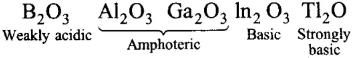(b) Nature of oxides of 15 group elements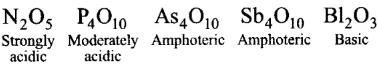Among the oxides of same element, higher the oxidation state of the element, stronger is the acid. For example, S03 is a stronger acid than S02.
B203 is weakly acidic and on dissolution in water, it forms orthoboric acid. Orthoboric acid does not act as a protonic acid (it does not ionise) but acts as a weak Lewis acid.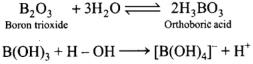Al2O3 is amphoteric in nature. It is insoluble in water but dissolves in alkalies and reacts with acids.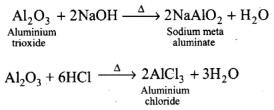Tl2O is as basic as NaOH due to its lower oxidation state (+1).
Tl20 + 2HCl → 2TlCl + H20
P4O10 on reaction with water gives orthophosphoric acid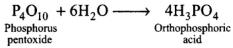Cl207 is strongly acidic in nature and on dissolution in water, it gives perchloric acid.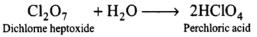Q.35. How would you explain the fact that first ionisation enthalpy of sodium is lower than that of magnesium but its second ionisation enthalpy is higher than that of magnesium?
Ans. Electronic configuration of Na is 1s22s22p63s1. After losing one electron from its outermost shell, sodium easily attains stable electronic configuration (1s22s22p), while magnesium does not lose its electron easily due to presence of two electrons in s-orbital (ls22s22p63s2). Hence first ionisation energy of sodium is less than magnesium.
When one electron is removed from Na and Mg, their configurations become ls22s22p6 and 1s22s22p63s1 respectively. Now it is easier to remove one electron from 3s of Mg+ than 2p6 of Na+. Hence, second ionisation energy of Mg is less than Na.

Q.36. What do you understand by exothermic reaction and endothermic reaction? Give one example of each type.
Ans. Exothermic reaction: The reaction which is accompanied with evolution of heat is known as exothermic reaction.
CaO + C02 → CaC03, ΔH= -178.3 kJ mol-1
Endothermic reaction: The reaction which is accompanied with absorption of energy is known as endothermic reaction, e.g.,
2NH3 → N2 + 3H2; ΔH = +91.8 kJ mol-1

Q.37. Arrange the elements N, P, O and S in the order of
(i) increasing first ionisation enthalpy.
(ii) increasing non metallic character.

Give reason for the arrangement assigned.
Ans. (i) S < P < O < N
Ionisation enthalpy increases from left to right in a period and decreases down the group. N has higher ionisation enthalpy than O due to extra stability of half-filled orbitals. Similarly, P has higher ionisation enthalpy than S due to half-filled orbitals.
(ii) P < S < N < O
Non-metallic character decreases down the group and increases from left to right in a period.

Q.38. Explain the deviation in ionisation enthalpy of some elements from the general trend by using Figure.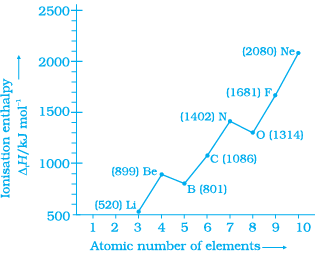Ans. Ionisation enthalpy increases in a period with increase in atomic number. The graph shows few exceptions and not the linear relationship. Ionisation enthalpy of Be is greater than that of B due to filled s-orbital in Be (Be – l.s1s2, B – 1s2 2s2 2pl).
Ionisation enthalpy of N is greater than that of O due to half-filled/?-orbitals in nitrogen.
(N – Is2 2s 22p3 O – ls2 2s2 2p4).

Q.39. Explain the following:
(a) Electronegativity of elements increase on moving from left to right in the periodic table.
(b) Ionisation enthalpy decrease in a group from top to bottom?
Ans.  (a) The electronegativity generally increases on moving across a period from left to right (e.g., from Li to F in the second period). This is due to decrease in atomic size and increase in effective nuclear charge. As a result of increase in effective nuclear charge, the attraction for the outer electron and the nucleus increases in a period and therefore, electronegativity also increases.
(b) On moving down a group there is a gradual decrease in ionisation enthalpy. The decrease in ionisation enthalpy down a group can be explained in terms of net effect of the following factors:
(i) In going from top to bottom in a group, the nuclear charge increases.
(ii) There is a gradual increase in atomic size due to an additional main energy shell (n).
(iii) There is increase in shielding effect on the outermost electron due to increase in the number of inner electrons.
The effect of increase in atomic size and the shielding effect is much more than the effect of increase in nuclear charge. As a result, the electron becomes less and less firmly held to the nucleus as we move down the group. Hence, there is a gradual decrease in the ionization enthalpies in a group.

Q.40. How does the metallic and non metallic character vary on moving from left to right in a period?
Ans.As we move from left to right in a period, the number of valence electrons increases by one at each succeeding element but number of shells remains same. Due to this, effective nuclear charge increases. More is the effective nuclear charge, more is the attraction between the nucleus and electron.
Hence, the tendency of the element to lose electrons decreases. This results in decrease in metallic character. Furthermore, the tendency of an element to gain electrons increases with increase in effective nuclear charge, so non- metallic character increases on moving from left to right in a period.

Q.41. The radius of Na+ cation is less than that of Na atom. Give reason.
Ans. The radius of Na+ is less than Na atom because Na+ is formed by losing one energy shell.
Na – 1s2 2s2 2p6 3s1
Na+– 1s22s2 2p6

Q.42. Among alkali metals which element do you expect to be least electronegative and why?
Ans. Cs is the most electropositive or least electronegative element among the alkali metals since electronegative character decreases in a group.

MATCHING TYPE

Q.43. Match the correct atomic radius with the element.

 Element Atomic radius (pm) Be 74 C 88 O 111 B 77 N 66

Ans. All the given elements are of same period and along period, atomic radii decrease because effective nuclear charge increases. Thus, the order of atomic radii is
O < N < C < B < Be or, Be = 111 pm, O = 66 pm, C = 77 pm, B = 88 pm, N = 74 pm.

Q.44. Match the correct ionisation enthalpies and electron gain enthalpies of the following elements.

 Elements ∆H1 ∆H2 ∆eg H (i) Most reactive non metal A. 419 3051 –  48 (ii) Most reactive metal B. 1681 3374 –  328 (iii) Least reactive element C. 738 1451 –  40 (iv) Metal forming binary halide D. 2372 5251 +  48

Ans. (i) → (b);
(ii) → (a);
(iii) → (d);
(iv) → (c)

Q.45. Electronic configuration of some elements is given in Column I and their electron gain enthalpies are given in Column II. Match the electronic configuration with electron gain enthalpy.

 Column (I) Column (II) Electronic configuration Electron gain enthalpy/kJ mol–1 (i) 1s2 2s2 sp6 (A) – 53 (ii) 1s2 2s2 2p6 3s1 (B) – 328 (iii) 1s2 2s2 2p5 (C) – 141 (iv) 1s2 2s2 2p4 (D) + 48

Ans. (i → D), (ii → A), (iii → B), (iv → C)
(i) This electronic configuration corresponds to the noble gas, i.e., neon. Since, noble gases have +ΔegH values, therefore, electronic configuration (A) corresponds to the ΔegH = +48 kJ mol-1.
(ii) This electronic configuration corresponds to the alkali metal, i.e., potassium. Alkali metals have small negative ΔegH values, hence, electronic configuration (B) corresponds to ΔegH  = -53 kJ mol-1.
(iii) This electronic configuration corresponds to the halogen, i.e., fluorine. Since, halogens have high negative  ΔegH values, therefore, electronic configuration (C) corresponds to  ΔegH = -328 kJ mol-1.
(iv) This electronic configuration corresponds to the chalcogcn, i.e., oxygen. Since, chalcogens have ΔegH values less negative than those of halogens, therefore, electronic configuration (D) corresponds to ΔegH = -141 kJ mol-1.

ASSERTION AND REASON TYPE QUESTIONS

In the following questions a statement of Assertion (A) followed by a statement of reason (R) is given. Choose the correct option out of the choices given below each question.
Q.46. Assertion (A) :
Generally, ionisation enthalpy increases from left to right in a period.
Reason (R) : When successive electrons are added to the orbitals in the same principal quantum level, the shielding effect of inner core of electrons does not increase very much to compensate for the increased attraction of the electron to the nucleus.
(i) Assertion is correct statement and reason is wrong statement.
(ii) Assertion and reason both are correct statements and reason is correct explanation of assertion.
(iii) Assertion and reason both are wrong statements.
(iv) Assertion is wrong statement and reason is correct statement.

Ans. (ii)
Solution.
Ionisation enthalpy increases from left to right across the period due to decrease in atomic size.
The electrons present within the subshell has almost same effective nuclear charge.

Q.47. Assertion (A) : Boron has a smaller first ionisation enthalpy than beryllium.
Reason (R) : The penetration of a 2s electron to the nucleus is more than the 2p electron hence 2p electron is more shielded by the inner core of electrons than the 2s electrons.
(i) Assertion and reason both are correct statements but reason is not correct explanation for assertion.
(ii) Assertion is correct statement but reason is wrong statement.
(iii) Assertion and reason both are correct statements and reason is correct explanation for assertion.
(iv) Assertion and reason both are wrong statement
s.
Ans. (iii)
Solution.
In beryllium, the electron removed during the ionization is an s-electron whereas the electron removed during ionization of boron is a p-electron. The penetration of a 2s-electron to the nucleus is more than that of a 2p-electron; hence the 2p electron of boron is more shielded from the nucleus by the inner core of electrons than the 2s electrons of beryllium. Therefore, it is easier to remove the 2p-electron from boron compared to the removal of a 2s-electron from beryllium. Thus, boron has a smaller first ionization enthalpy than beryllium.

Q.48. Assertion (A) : Electron gain enthalpy becomes less negative as we go down a group.

Reason (R) : Size of the atom increases on going down the group and the added electron would be farther from the nucleus.
(i) Assertion and reason both are correct statements but reason is not correct explanation for assertion.
(ii) Assertion and reason both are correct statements and reason is correct explanation for assertion.
(iii) Assertion and reason both are wrong statements.
(iv) Assertion is wrong statement but reason is correct statement.
Ans. (ii)
Solution.
On moving down the group, the electron gain enthalpy becomes less negative because on moving down the group the atomic size increases and the added electron lies away from the nucleus.

Q.49. Discuss the factors affecting electron gain enthalpy and the trend in its variation in the periodic table.
Ans.
Factors affecting electron gain enthalpy:
(i) Nuclear charge: The electron gain enthalpy become more negative as the nuclear charge increases. This is due to greater attraction for the incoming electron if nuclear charge is high.
(ii) Size of the atom: With the increase in size of the atom, the distance between the nucleus and the incoming electron increases and this results in lesser attraction. Consequently, the electron gain enthalpy become less negative with increase in size of the atom of the element.
(iii) Electronic configuration: The elements having stable electronic configurations of half filled and completely filled valence subshells show very small tendency to accept additional electron and thus electron gain enthalpies are less negative.
Variation of electron gain enthalpies in periodic table:
Electron gain enthalpy, in general, becomes more negative from left to right in a period and becomes less negative as we go from top to bottom in a group.
(a) Variation down a group: On moving down a group, the size and nuclear charge increases. But the effect of increase in atomic size is much more pronounced than that of nuclear charge and thus the additional electron feels less attraction by the large atom. Consequently, electron gain enthalpy becomes less negative. This is clear from decrease of electron gain enthalpy in going from chlorine to bromine and to iodine.
(b) Variation along a period: On moving across a period, the size of the atom decreases and nuclear charge increases. Both these factors result in greater attraction for the incoming electron, therefore, electron gain enthalpy, in general, becomes more negative in a period from left to right. However, certain irregularities are observed in the general trend. These are mainly due to the stable electronic configurations of certain atoms.
Important Trends in Electron Gain Enthalpies:
There are some important features of electron gain enthalpies of elements.
These are:
(i) Halogens have the highest negative electron gain enthalpies.
(ii) Electron gain enthalpy values of noble gases are positive while those of Be, Mg, N and P are almost zero.
(iii) Electron gain enthalpy of fluorine is unexpectedly less negative than that of chlorine.

Q.50. Define ionisation enthalpy. Discuss the factors affecting ionisation enthalpy of the elements and its trends in the periodic table.
Ans. Ionisation enthalpy: It is defined as the minimum amount of energy required to remove the most loosely bound electron from an isolated gaseous atom.
M(g) →M+(g) + eI1  = First ionisation enthalpy
Similarly, second and third electrons are also removed by providing successive ionisation enthalpies.
Factors on which Ionisation Enthalpy Depends:
(i) Size of the atom: The larger the atomic size, smaller is the value of ionisation enthalpy. In a larger atom, the outer electrons are far away from the nucleus and thus force of attraction with which they are attracted by the nucleus is less and hence can be easily removed.
Ionisation enthalpy ∝ 1/ Atomic size
(ii) Screening effect: Higher the screening effect, the lesser is the value of ionisation enthalpy as the screening effect reduces the force of attraction towards nucleus and hence the outer electrons can be easily removed.
Ionisation enthalpy ∝  1/ Screening effect
(iii) Nuclear charge: As the nuclear charge increases among atoms having same number of energy shells, the ionisation enthalpy increases because the force of attraction towards nucleus increases.
Ionisation enthalpy ∝ Nuclear charge
(iv) Half filled and fully filled orbitals: The atoms having half filled and fully filled orbitals are comparatively more stable, hence more energy is required to remove the electron from such atoms. The ionization enthalpy is rather higher than the expected value in case of such an atom.
Ionisation enthalpy ∝ Stable electronic configuration
(v) Shape of orbital: The s-orbital is more close to nucleus than the p-orbital of the same orbit. Thus, it is easier to remove electron from a p-orbital in comparison to s-orbital. In general, the ionisation enthalpy follows the following order
(s>p> d>f) orbitals of the same orbit.
Variation of ionisation enthalpy in the periodic table
ln general, the ionisation energy decreases down the group due to increase in atomic size. On the other hand, the ionisation energy increases across the period from left to right, again due to decrease in atomic size from left to right.

Q.51. Justify the given statement with suitable examples— “the Properties of the elements are a periodic function of their atomic numbers”.
Ans. The physical and chemical properties of the elements are periodic functions of their atomic numbers. Periodic Law is essentially the consequence of the periodic variation in electronic configurations, which indeed determine the physical and chemical properties of elements and their compounds. Elements having similar outer electronic configurations in their atoms are arranged in vertical columns, referred to as groups or families.

Q.52. Write down the outermost electronic configuration of alkali metals. How will you justify their placement in group 1 of the periodic table?
Ans.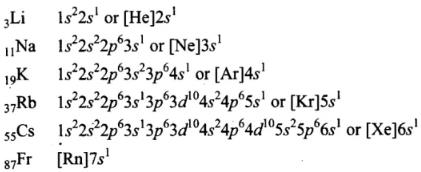Since all the elements have one electron in their valence shell, hence, they are placed in first group of the periodic table.

Q.53. Write the drawbacks in Mendeleev’s periodic table that led to its modification.
Ans. Drawbacks of Mendeleev’s periodic table:
1. Position of hydrogen: Hydrogen is placed in group I. However, it resembles the elements of group I (alkali metals) as well as the elements of group VILA, (halogens). Therefore, the position of hydrogen in the periodic table is not correctly defined.
2. Anomalous pairs: In certain pairs of elements, the increasing order of atomic masses was not obeyed. In these cases, Mendeleev placed elements according to similarities in their properties and not in increasing order of their atomic masses. For example, argon (Ar, atomic mass 39.9) is placed before potassium (K, atomic mass 39.1). Similarly, cobalt (Co, atomic mass 58.9) is placed before nickel (Ni, atomic mass 58.6) and tellurium (Te, atomic mass 127.6) is placed before iodine (I, atomic mass 126.9). These positions were not justified.
3. Position of isotopes: Isotopes are the atoms of the same element having different atomic masses but same atomic number. Therefore, according to Mendeleev’s classification, these should be placed at different places depending upon their atomic masses. For example, isotopes of hydrogen with atomic masses 1,2 and 3 should be placed at three places. However, isotopes have not been given separate places in the periodic table.
4. Some similar elements are separated and dissimilar elements are grouped together: In the Mendeleev’s periodic table, some similar elements were placed in different groups while some dissimilar elements had been grouped together. For example, copper and mercury resembled in their properties but they had been placed in different groups. At the same time, elements of group IA such as Li, Na and K were grouped with copper (Cu), silver (Ag) and gold (Au), though their properties are quite different.
5. Cause of periodicity: Mendeleev did not explain the cause of periodicity among the elements.
6. Position of lanthanoids (or lanthanides) and actinoids (or actinides): The fourteen elements following lanthanum (known as lanthanoids, from atomic number 58-71) and the fourteen elements following actinium (known as actinoids, from atomic number 90 – 103) have not been given separate places in Mendeleev’s table.
In order to cover more elements, Mendeleev modified his periodic table.

Q.54. In what manner is the long form of periodic table better than Mendeleev’s periodic table? Explain with examples.
Ans. Superiority of the Long form of the Table over Mendeleev’s Table:
(i) This table is based on a more fundamental property, i.e., atomic number.
(ii) It correlates the position of the elements with their electronic configurations more clearly.
(iii) The completion of each period is more logical. In a period as the atomic number increases, the energy shells are gradually filled up until an inert gas configuration is reached. It eliminates the even and odd series of IV, V, VI and VII periods of Mendeleev’s periodic table.
(iv) The position of VIIIth group  is appropriate in this table. All the transition elements have been brought in the middle as the properties of transition elements are intermediate between s- and p-block elements.
(v) Due to separation of two subgroups, dissimilar elements do not fall together. One vertical column accommodates elements with same electronic configuration thereby showing same properties.
(vi) In this table, a complete separation between metals and non-metals has been achieved. The non-metals are present in the upper right comer of the periodic table.
(vii) There is a gradual change in properties of the elements with increase in their atomic numbers, i.e., periodicity of properties can be easily visualized. The same properties o recurrence in properties occur after the intervals of 2, 8, 8, 18, 18 and 32 elements which indicates the capacity of various periods of the table.
(viii) This arrangement of elements is easy to remember and reproduce.

Q.55. Discuss and compare the trend in ionisation enthalpy of the elements of group 1 with those of group 17 elements.
Ans. The ionization enthalpies decrease regularly as we move down a group from one element to the other. This is evident from the values of the first ionisation enthalpies of the elements of group 1 (alkali metals) and group 17 elements as given in table and figure.

 Group 1 First ionisation enthalpies (kJ moL-1) Group 17 First ionisation enthalpies (kJ mol-1) H 1312 F 1681 Li 520 Cl 1255 Na 496 Br 1142 K 419 I 1009 Rb 403 At 917 Cs 374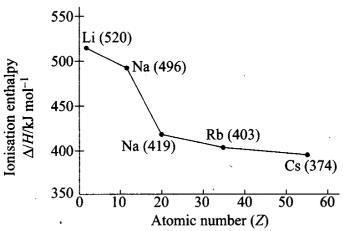Given trend can be easily explaihed on the basis of increasing atomic size and screening effect as follows
(i) On moving down the group, the atomic size increases gradually due to the addition of one new principal energy shell at each succeeding element. Hence, the distance of the valence electrons from the nucleus increases.
Consequently, the force of attraction by the nucleus for the valence electrons decreases and hence the ionisation enthalpy should decrease.
(ii) With the addition of new shells, the shielding or the screening effect increases. As a result, the force of attraction of the nucleus for the valence electrons further decreases and hence the ionisation enthalpy should decrease.
(iii) Nuclear charge increases with increase in atomic number. As a result, the force of attraction by the nucleus for the valence electrons should increase and accordingly the ionisation enthalpy should increase.
The combined effect of the increase in the atomic size and the screening effect more than compensates the effect of the increased nuclear charges. Consequently, the valence electrons become less and less firmly held by the nuclear and hence the ionisation enthalpies gradually decrease as move down the group.

Offer running on EduRev: Apply code STAYHOME200 to get INR 200 off on our premium plan EduRev Infinity!

233 docs

,

,

,

,

,

,

,

,

,

,

,

,

,

,

,

,

,

,

,

,

,

;